arrow_back_iosQuestions
help

# Customers can book for any number of days, but our prices depends on the number of reserved weeks and months. Is this supported?

check_circle
In this case, you can set the cost using pricing components.
Create a separate pricing component for each duration. For example: 1 week, 2 weeks, 3 weeks, etc. In each component, limit it to the appropriate number of days.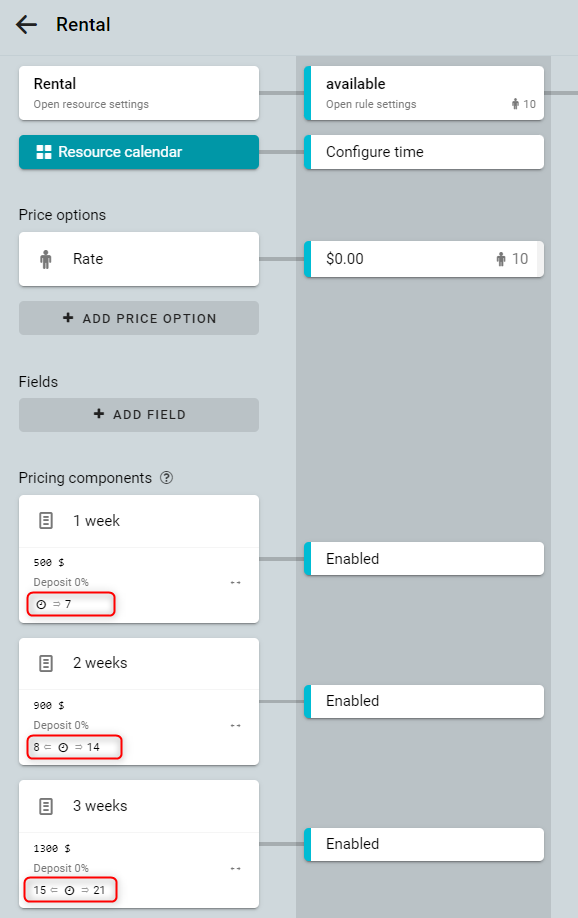Set the price in the formula and click on the Add condition button.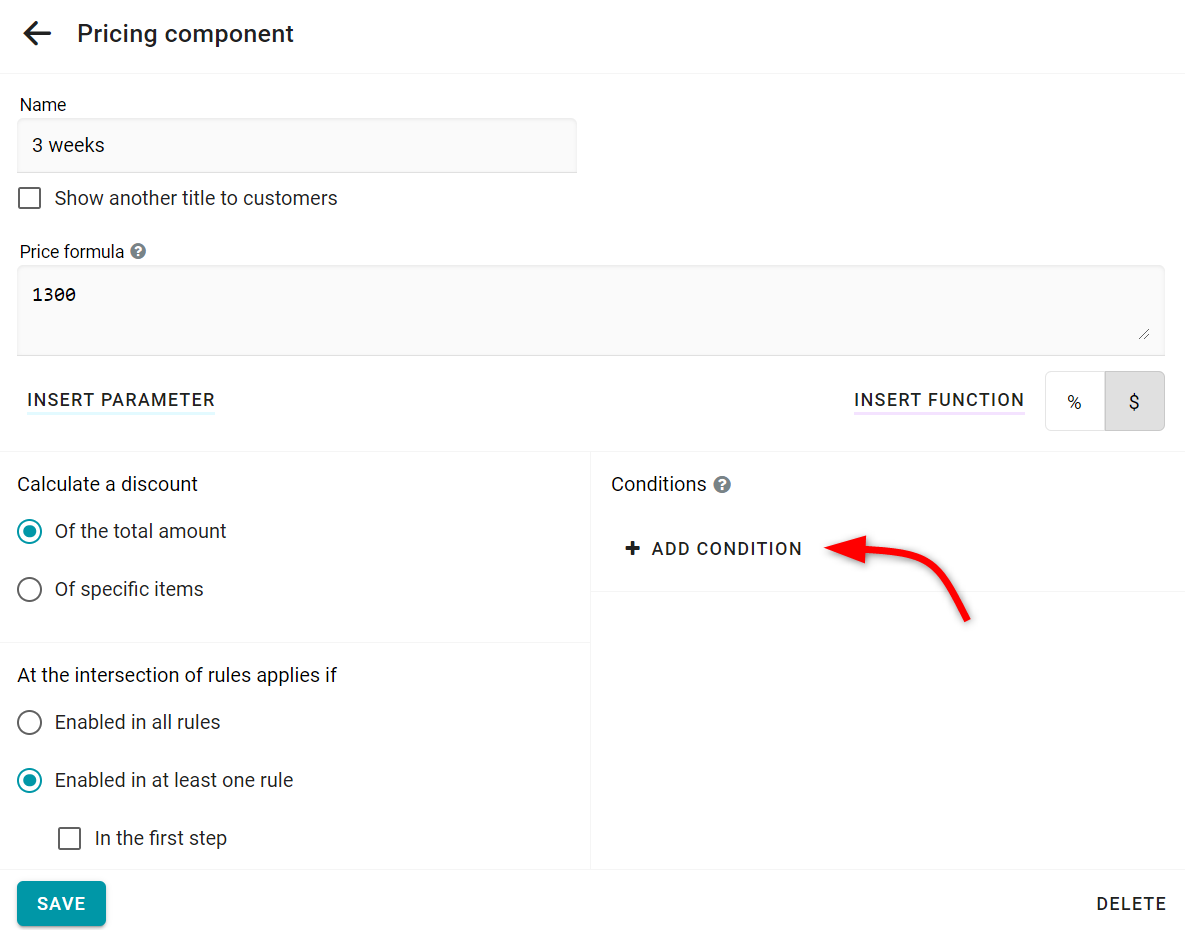Then pick the Duration option (a number of booked days) and specify Minimum steps and Maximum steps.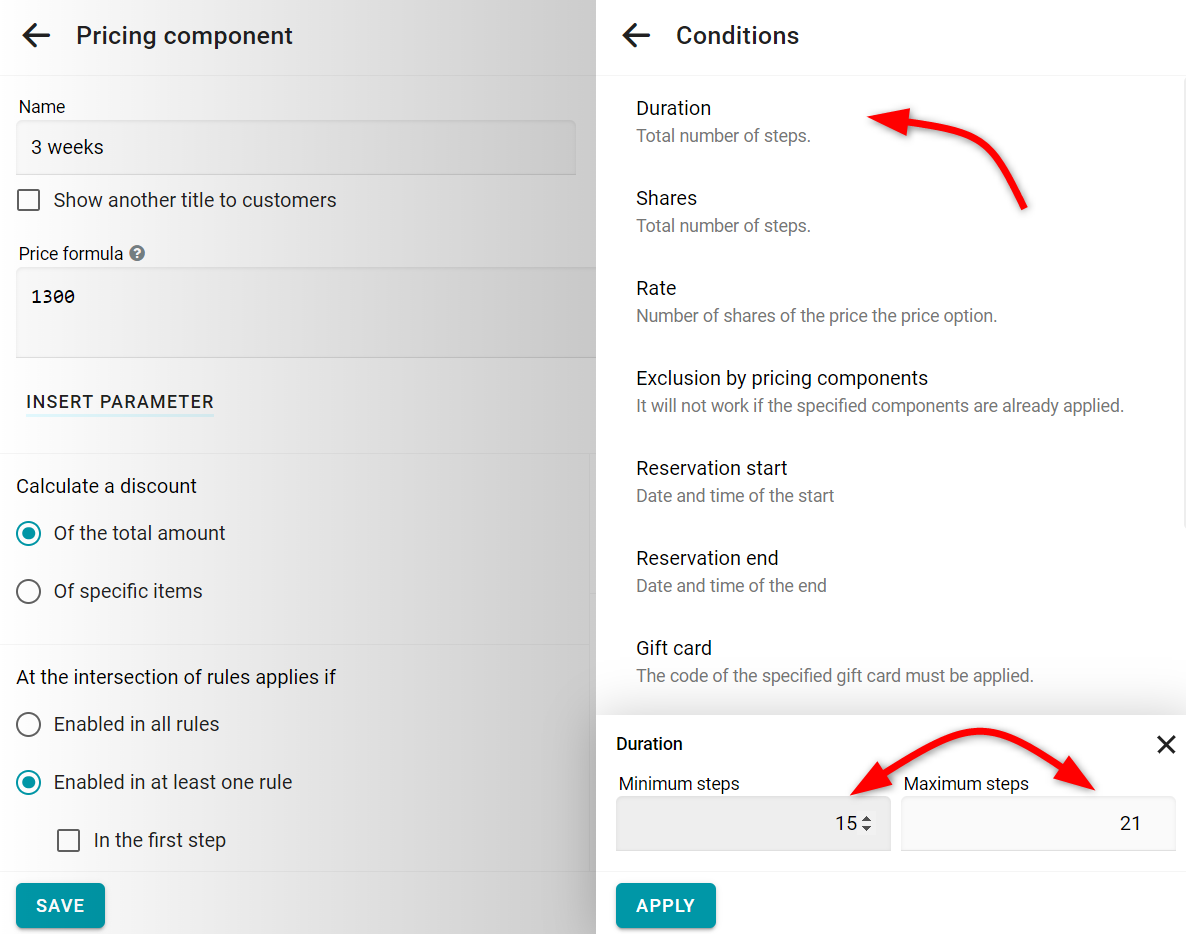Alternatively, you can set a single formula based on the number of days booked.
For example, the cost is \$1000 / month.
The formula for month price calculation looks like this: 1000 * Ceiling([STEPS]/31)
\$1000 is multiplied by the number of booked days that are divided by 31 (a number of days in a month). Since the months are different, there may be inaccuracies of several days for long bookings.
In this formula, we used the Ceiling function. It rounds the number up. In order to add a function click on the Insert function button.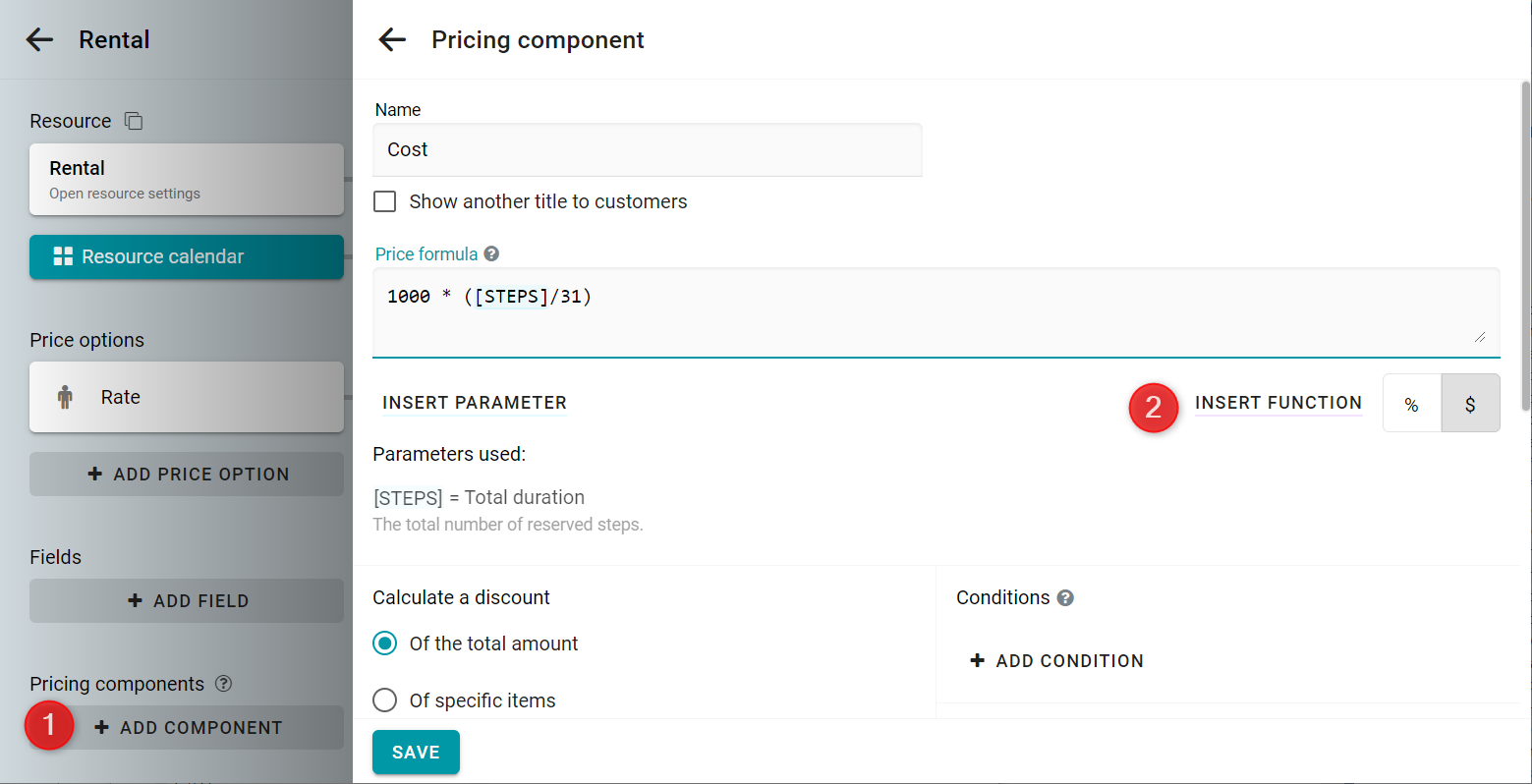Then pick Ceiling from the list of functions.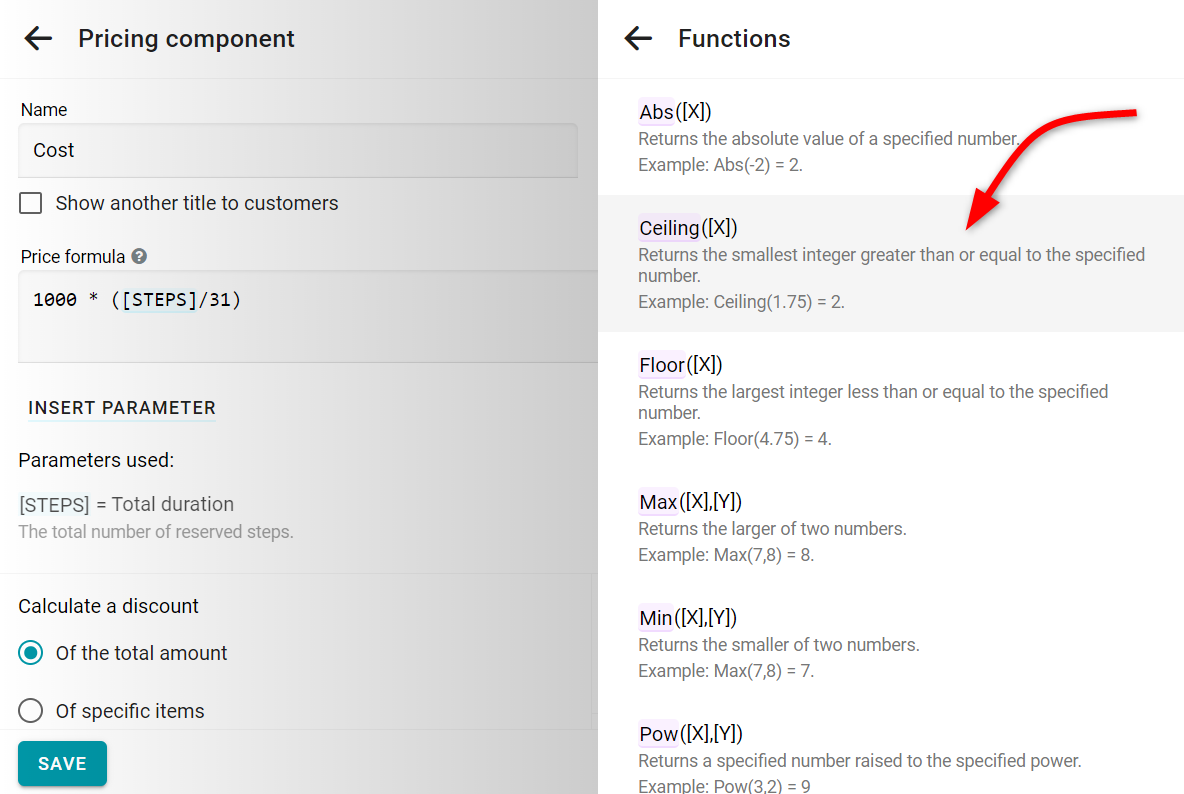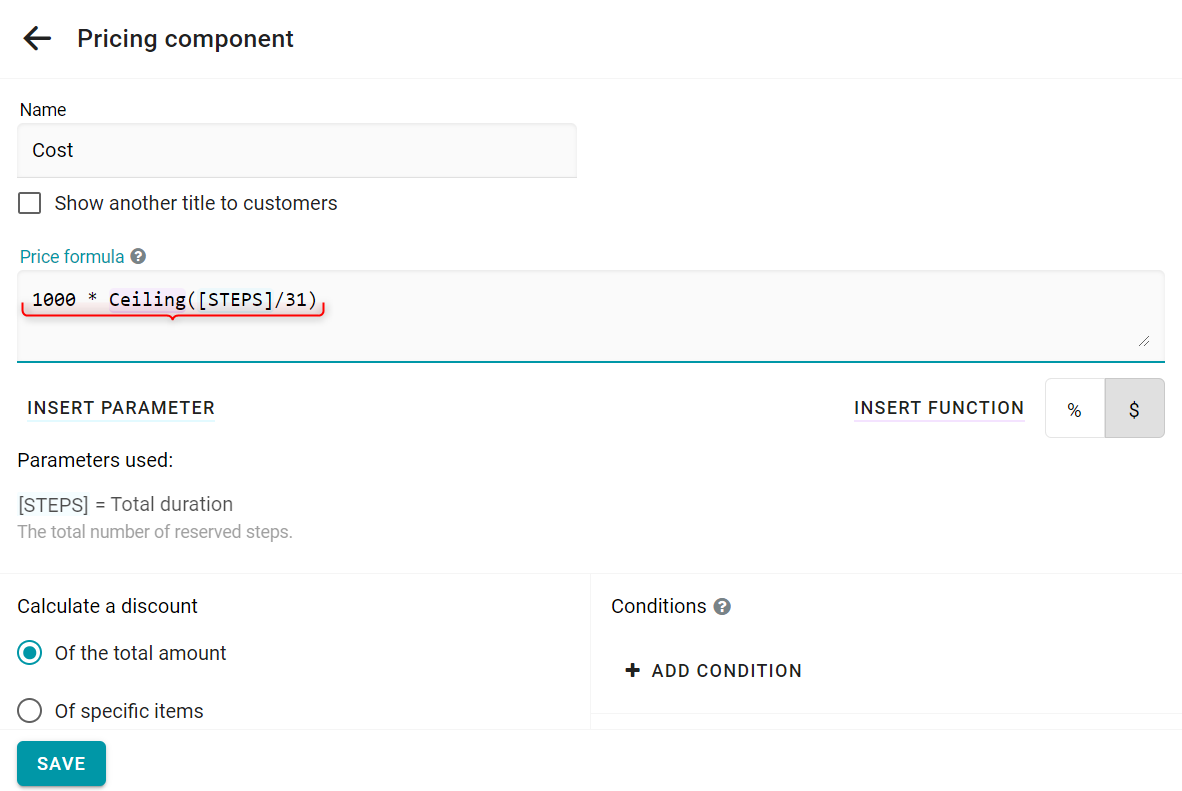For the formula of weeks, simply replace 31 with 7.
See this example in action: